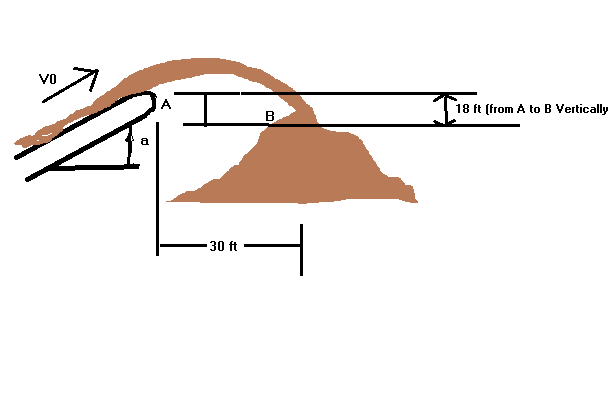# Another dynamics problem involving projectile kinematics

Khamul

## Homework Statement

Sand is discharged at A from a conveyor belt and falls onto the top of a stockpile at B. Knowing that the conveyor belt forms an angle $$\alpha$$= 20 degrees with the horizontal, determine the speed V0 of the belt.

## Homework Equations

xf-xi=Vi,x*t
yf-yi=Vi,y*t - (1/2)(g)(t2)
Vi,x=Vi*cos($$\theta$$)
Vi,y=Vi*sin($$\theta$$)

## The Attempt at a Solution

Hello again guys! I know this problem is very similar to the last one that had the snowballs, the only difference being that there is now an upwards 20 degree angle instead of it being flatly horizontal.

Using the values I could relate Vi to, I found the numerical value of the angle * Vi, and plugged the respective ones into the vertical and horizontal equations. I then found in the horizontal equation, what Vi equaled. After this, I plugged the Vi relation into the vertical equation, solved the time( I got t= 2.428 s ) and plugged the information in. I found the initial velocity to be 13.15 m/s...but the answer in the book says it is 23.8 ft/s.

Would anyone be so kind as to help me out with where I went wrong? Do I need to use the same equation I used for the vertical component as I do for the horizontal? Tieing into that question, does this angle mean that there is an acceleration in the horizontal component as well?

Thanks for any replies!

4. F.B.D.
Sorry it's really crude, but it illustrates everything nicely I believe.## Answers and Replies

Homework Helper
Hi Khamul!(have a theta: θ)

## Homework Equations

xf-xi=Vi,x*t
yf-yi=Vi,y*t - (1/2)(g)(t2)
Vi,x=Vi*cos($$\theta$$)
Vi,y=Vi*sin($$\theta$$)

Those are the correct formulas.

Show us your full equations, so that we can see what's going wrong.Khamul
Hi Khamul!(have a theta: θ)

Those are the correct formulas.

Show us your full equations, so that we can see what's going wrong.Hello again, Tiny! Hooray, a θ!And, alright--sorry if it gets a bit messy but i'll try and explain!

First, I set the x and y components of the initial velocity equal to the velocity times the appropriate trig/angle values:
Vi,x=Vi*cos(20) ---> Vi,x= Vi*(.9397)
Vi,y=Vi*sin(20) ---> Vi,y= Vi*(.3420)

After I found those, and knowing the x final and initial values were 30ft and 0ft respectively; and that the y initial and final values were 0ft and -18ft respectively, I created two equations:

-Vertical Component:
(yf-yi)=Vi,y*t - (1/2)*g*t2
--> -18ft=Vi*(.3420)*t-4.905t2

-Horizontal Component:
(xf-xi)=Vi,x*t
-->30=Vi*(.9397)*t

After this, I rearranged the horizontal component equation to:
30/[(.9397)*t] = Vi

I then plugged the Vi value into the vertical component equation, yielding;
-18ft=[30(.342)*t]/[.9397*t] - 4.905*t2.

The t's cancel, and simplifying further I got:
-18=10.918-4.905*t2
-->t2=5.896
--->t=2.428 s

After finding this value, I went back to both horizontal and vertical equations and subbed in my value for time, and I got Vi=13.14 ft/s.

And like I said..my book is claiming the answer is 23.8 ft/s though. Is it possible that my book is incorrect, or did I make a large blunder somewhere?Homework Helper
Hello Khamul!-Vertical Component:
(yf-yi)=Vi,y*t - (1/2)*g*t2
--> -18ft=Vi*(.3420)*t-4.905t2

erm… g is 32Khamul
Hello Khamul!erm… g is 32AHHHH! I can't believe I missed that, I was even going back checking units!:shy: Thanks tiny-tim!....time for me to go facedesk for awhile.